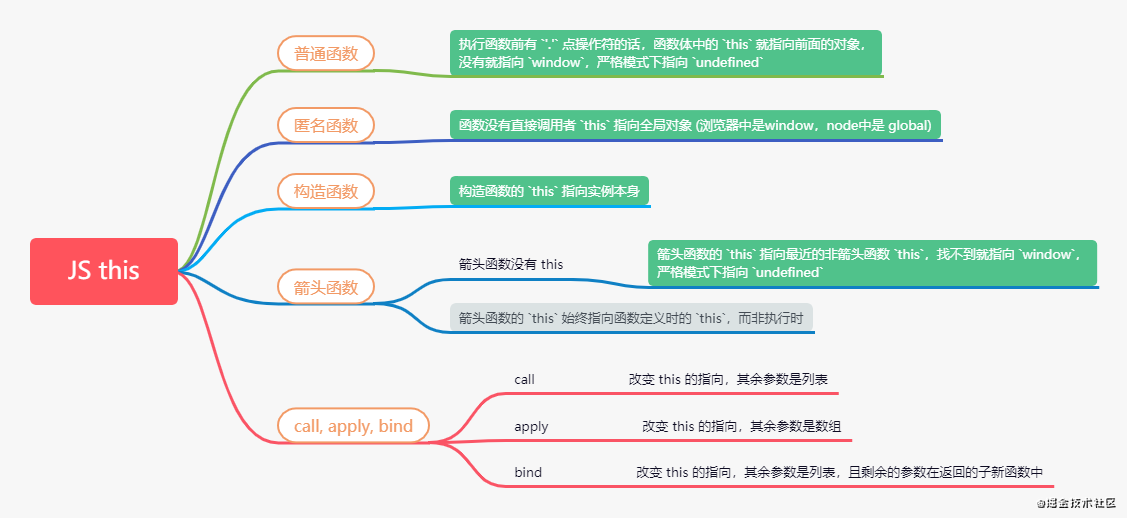# 面试 | 你不得不懂的 JS this 指向

epeppanda

0收藏this 的指向

``````var name = '林一一'
function fn(){
var name = '林二二'
return this.name
}
fn()    // 林一一
``````

``````var name = '林二二'
var obj = {
name: '林一一',
fn: function () {
return this.name
}
}
console.log(obj.fn())   // '林一一'
var fo = obj.fn
console.log(fo())       // '林二二'     fo() ==> window.fo()
``````

obj.fn() 中函数 fn() 前面有 '.' 点操作符吧，那么这里的 this 就指向 obj 这个对象。再看执行函数 fo()，前面没有 '.' 点操作符吧，那么这里的 this 就指向 window。其实上面的函数 fo() ==> window.fo()，所以执行函数 fo() 前面也是可以看作是有 '.' 操作符的。

``````var name = '林二二'
var obj = {
name: '林一一',
fn: function () {
var name = '小三'
return function(){
return this.name
}
}
}
console.log(obj.fn()())   // 林二二
var fo = obj.fn()
console.log(fo())    // 林二二
``````

``````var name = '林一一';
!(function(){
console.log(this.name)   // 林一一
})()
``````

``````var name = '林一一'
var obj = {
name : '二二',
callback: function(){
console.log(this.name)
}
}

setTimeout(obj.callback,1000)
/* 输出
*   林一一
*/
``````

``````function Fn(){
var n = 0
this.name = '林一一'
this.age = 18
this.getName = function(){
return this.name
}
}

Fn.prototype.getAge = function(){
return this.age
}

Fn.x = '林二二'

var f = new Fn()
console.log(f.name)     // 林一一
console.log(f.getName())     // 林一一
console.log(f.getAge())        // 18
console.log(f.n)    // undefined
console.log(f.x)    // undefined
``````

``````var name = '林一一'
var obj = {
name: '二二',
a: () => {
console.log(this.name)
}
}
obj.a()

/* 输出
*   '林一一'
*/
``````

``````var name = '林一一'
var obj = {
name: '二二',
fn: function() {
return () => {
console.log(this.name)
}
}
}
obj.fn()()

/* 输出
*   '二二'
*/
``````

call，接受this的对象，和一组列表。apply 和 call 一样，唯一不同的是 apply 接受的是一个包含多个参数的数组。bind 同样也是改变函数的 this 指向，只不过 bind 执行后会返回一个新的函数，新函数中参数来源于剩余的参数

``````var name = '林一一'
var age = 18
function fn(){
return this.name
}

function p(){
return {
age: this.age,
arg: arguments
}
}

let obj = {
name: '二二',
age: 18
}

let o = {
name: '三三'
}

fn()    // '林一一'
fn.call(obj)    // '二二'
fn.call(o)  //  '三三'
p.call(obj, 12, 23, 45, 67) // {age: 18, arg: Arguments(4)}

fn.apply(obj)    // "二二"
p.apply(obj, [1, 2, 3, 4, 5])    // {age: 18, arg: Arguments(5)}

fn.bind(obj)()  // "二二"
p.bind(obj, 12, 23, 34)()   // {age: 18, arg: Arguments(3)}
``````

1. 笔试题 this 指向问题

``````var name = '林一一'
var obj = {
name: '林二二',
show: function (){
console.log(this.name)
},
wait: function () {
var fn = this.show
fn()
}
}
obj.wait()  //  林一一
``````

obj.wait() 中，执行函数 wait() 前面有 '.' 点操作符吧，那么这里的 this 就指向 obj 这个对象，所以this.show ==> obj.show。再看执行函数 fn() 前面没有 '.' 点操作符吧，那么这里的 this 就指向 window，输出就是 林一一。

2. 阿里 this 指向和原型面试题（新增）

``````function Foo() {
Foo.a = function() {
console.log(1)
}

this.a = function() {
console.log(2)
}
}

Foo.prototype.a = function() {
console.log(3)
}

Foo.a = function() {
console.log(4)
}

Foo.a();

let obj = new Foo();
obj.a();
Foo.a();
/*
*   4
*   2
*   1
*/
``````

Foo.a(); 中直接调用函数的私有方法a 输出结果就是 4。new Foo(); 过程中函数的私有 a 被重新赋值，同时原型prototype 上的属性a也被重新赋值。所以obj.a(); 输出结果就是 2，Foo.a();输出结果就是 1。

3. 和闭包有关的 this 指向问题

``````var n = 2 // -> 4 -> 8
var obj = {
n: 3,    // 6
fn: (function(n){
n*=2
this.n+=2    // window 下的 n 变成 4
var n = 5    // 这一步不会再重新声明，因为已经参数赋值，就不会再声明而是直接赋值 n = 5
console.log("window.n", window.n)
return function (m) {
console.log("n:", n, "m", m)    // n:5  m:3  这里的 n 向上查找是 5   //
this.n*=2    // fn(3): 2 * 4 =8  //  obj.fn(3): 2 * 3 = 6
console.log(m + (++n))    // 3 + (++5) ++n 导致上级作用域的n变成了6    // 3 + (++6)
}
})(n)
}

var fn = obj.fn;
fn(3)    // 9
obj.fn(3)    // 10
console.log(n, obj.n)    // 8 6
/* 输出
* 9
* 10
* 8  6
/
``````帖子
视频
声望
粉丝
最近发布
社区精华内容